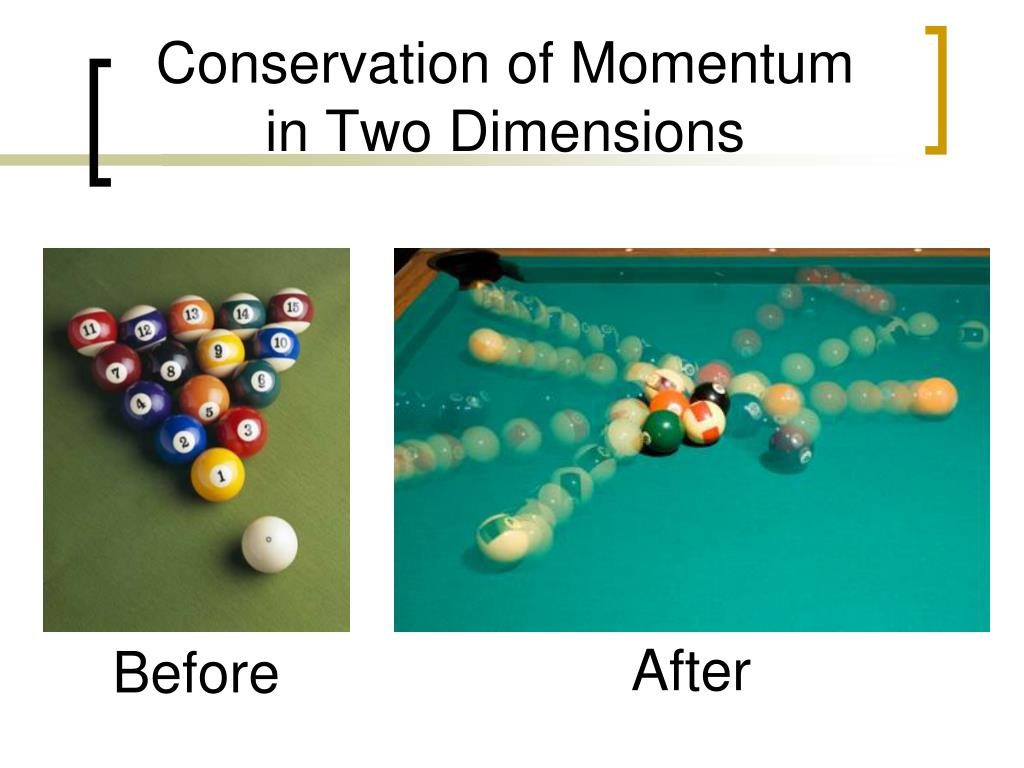Crystal

MS-PS2-2

# Conservation Of Momentum In Two Dimensions Study Guide

In this chapter, we go deeper into the conservation of momentum in two dimensions. Since momentum is a vector quantity, it can be represented by axial-vector components.

# IntroductionSource

The collision between two objects in the real world seldom occurs in only one direction. Consider the game of billiards; it is critical that the shooter can visualize the direction in which the colored balls take off when struck by the cue ball. While the players might get the shot right by instinct and practice, there is precise physics that governs how the billiard balls behave. To know how this works, it is essential to understand the conservation of momentum in two dimensions.

## THE PRINCIPLE OF CONSERVATION OF MOMENTUM

Understanding the principle of conservation of momentum is essential before we can grasp how collisions work. In all closed systems, the total momentum of all colliding objects remains the same before and after the collision.

• By definition, a closed system is a system that is isolated from its surroundings such that it cannot exchange energy, mass, or work in any form.
• In the real world, such a system doesn’t exist.
• However, a pool table is a close approximation if we can ignore the small amounts of energy lost by the balls because of the friction of the table and air resistance.

According to the law of conservation of momentum, the total momentum of the balls before the collision is equal to the total momentum of the balls after the collision.

Mathematically the general equation in one dimension can be expressed as:

Σm1v1 = Σm2v2

Where, Σm1v1 is the sum total of momentum of all the balls before the collision

Σm2v2 is the sum total of momentum of all the balls after the collision

This equation gives the momentum relation when the balls collide head-on and move back in the same direction they came from.

## CONSERVATION OF MOMENTUM PRINCIPLE IN TWO DIMENSIONSSource

On a pool table, the collisions and movement of the balls rarely occur in one dimension. Most often, the balls graze one another and strike at different angles. Even when balls strike at different angles, the total momentum is still conserved.

Here we can apply the conservation of momentum law in two dimensions to account for the x and y components of motion. In this case, the momentum in each direction is conserved independently.

Mathematically it can be expressed as: Σpxi = Σpxf (total momentum in the x-direction before and after collision)

Σpyi = Σpyf (total momentum in the y-direction before and after collision)

# SUMMARY

• The law of conservation of momentum holds good for all closed systems.
• In a two-dimensional system, the momentum is conserved individually for each dimension because momentum is a vector quantity.

## FAQs

Q. What is the law of conservation of momentum in two dimensions?
In a closed system, when objects moving in two dimensions (x and y) collide, the momentum is conserved in each dimension individually.

Q. Is momentum conserved in each dimension?

According to the conservation of momentum law in two dimensions, the momentum will be conserved independently in each direction.

Q. What does conservation of momentum depend on?

The conservation of momentum is a consequence of Newton’s third law which states that every action has an equal and opposite reaction. It is also essential that the system being analyzed is closed or comes close to being isolated. The conservation of momentum cannot hold well if there is an external impulse on the objects or a significant loss of energy in the form of inelastic collisions.

Q. Why can momentum be conserved when energy is not?

As long as the system is isolated, both the momentum and energy are conserved.

We hope you enjoyed studying this lesson and learned something cool about the Conservation of Momentum in Two Dimensions! Join our Discord community to get any questions you may have answered and to engage with other students just like you! We promise, it makes studying much more fun!😎

1. Conservation of Momentum in Two-dimension: https://flexbooks.ck12.org/cbook/ck-12-physics-flexbook-2.0/section/6.3/primary/lesson/conservation-of-momentum-in-two-dimensions-phys/ Accessed 13th April 2022.
2. What are Two-dimensional Collisions? https://www.khanacademy.org/science/physics/linear-momentum/momentum-tutorial/a/what-are-two-dimensional-collisions#:~:text=For%20a%20collision%20where%20objects,before%20and%20after%20the%20collision. Accessed 13th April 2022.
3. Collisions of Point Masses in Two-dimension: https://courses.lumenlearning.com/physics/chapter/8-6-collisions-of-point-masses-in-two-dimensions/ Accessed 13th April 2022.